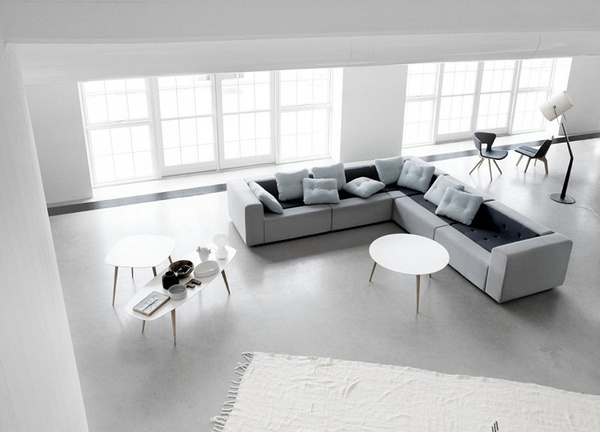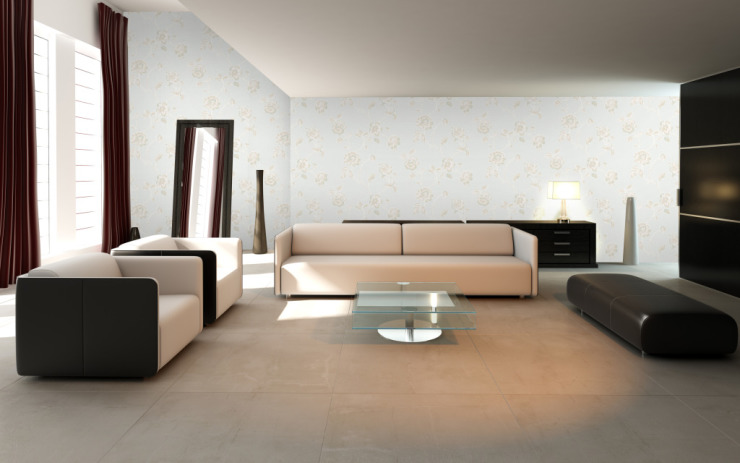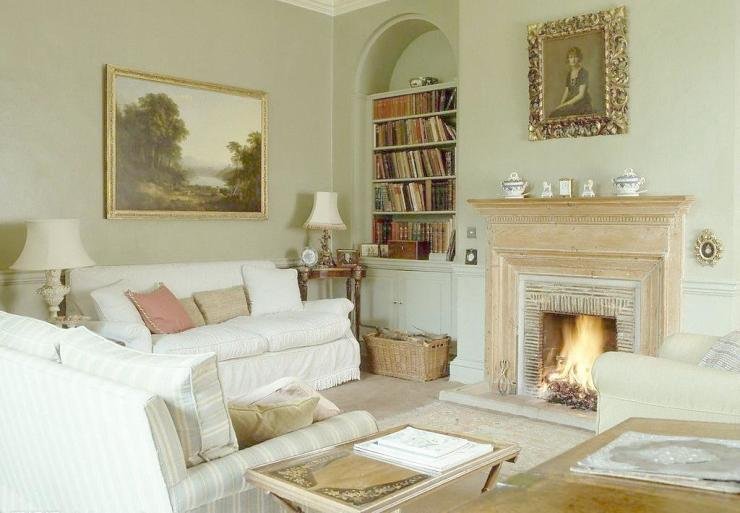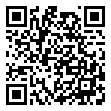|

# 你听说的新家风水可不只是迷信,现在都有了科学的论据

人人都急着想给自己的家换上新装束的时候，小编提醒大家，千万要注意房间的风水问题，别满心欢喜地布置了一个漂漂亮亮的新家，却犯上了风水大忌，那可冲了新房的喜气，给之后的日子带来晦气。

禁忌1：中心受污

释义：指房屋的中心部位不宜用作厕所。

风水说法：房屋的中心部位如果用作厕所，便有如人的心脏堆积废物，主人凶多吉少，易生病。如果厕所刚巧是在房屋的正中部位，那便不宜选作居所。倘若厕所并不是位于房屋中心，但却位于房屋后半部的中心，刚好与大门成一直线，亦不宜选作居所，可能导致破财损丁。科学揭秘：事实上，布局合理的户型设计中，厕所应该都有一个外窗，就是为了便于污气排放。但是厕所如果在房屋中心位置，空气则不易流通。一方面厕所里所释放的臭气会影响居住者的心情，另一方面排泄物中可能含有对人体有害的成分，主人容易生病。

禁忌2：户门三忌

释义：房间的正门即户门不宜正对厨房、主卧、厕所。

风水说法：开门忌见灶，“开门见灶，钱财多耗。”即入门见到灶，火气冲人，令财气无法进入。开门忌见厕，一进大门就见到厕所，则犹如秽气迎人。开门忌见榻，进户门正对着主人的卧室，主人多凶险，不利于聚财。科学揭秘：家中的空气比外界洁净，而且从外面进屋之后身上难免携带各种细菌。如果大门正对着厨房，开门的气流就有可能把身上的细菌吹到厨房里面，厨房是做饭的地方，所以如果长期保持这种布局，主人容易患呼吸道和食道疾病。而如果户门正对着主卧，不仅不利于卧室空气清洁，更重要的是，万一遇到歹徒进屋，第一时间受到伤害的就是房屋主人。

禁忌3：床边空虚

释义：“床边空虚”是指床放在房屋的中央位置，或四周空旷，不依靠墙壁。

风水说法：床适合摆放在卧室的角落里，靠墙的变数越多越适合，因为房间角落斜对房门，是屋子里面最能藏风聚气的地方，有利于主人的事业和财运。科学揭秘：从习惯上来说，人平躺时不容易看见头前的情况，所以床头宜靠墙、避免露空而减少安全感。特别是床头空虚，潜意识里便会缺乏安全感，以致夜间多梦，睡眠质量降低，日间容易有精神不振等情况出现。

禁忌4：床头靠窗

释义：床头放置在床下或者靠窗一侧的墙壁。

风水说法：窗户为理气进出之所，所以床头贴靠窗口容易犯冲。在睡床上的人因看不见头前的窗口，容易缺乏安全感，造成精神紧张，影响健康。科学揭秘：窗户就像房间的眼睛，特别是夜间睡觉时，屋外光线暗，而屋内明亮。或平躺或背靠，身处在这样的环境下会感觉到自己被眼睛盯着，而自己完全看不到这个危险之处的变化，引起心神不宁。另一方面，窗口是一个气流和光线最强的地方，动象很大，对睡眠影响很大。人的能量容易散失，因此对身体的健康也非常不利。

禁忌5：座位背窗

释义：家里的办公桌或者书桌不宜背靠门窗放置，应该背靠墙壁。

风水说法：从风水学的角度来看，好风水的第一大原则是“山环水抱”，所以座位背后必须要有靠山才有利于工作者的事业。办公室里的所谓“靠山”就是一堵墙壁，座位要尽量靠着墙壁，墙壁与座位之间最好不要留太多的空间。门是人进出的必经之处，门窗都是办公室的气口，也是纳气之所，包括生气和煞气。人如果背着门口而坐，座位后没有依靠，背后会有人来人往的杂气冲击。科学揭秘：事实上，如果背靠经常过人的门窗，在工作中就会经常听到行人的脚步声、喧哗声，以及其他的噪声干扰自己的工作，而且还容易把自己的工作过程甚至工作机密暴露于别人的失业中。还有可能被突如其来的声音或者恶作剧惊吓。即使是高楼的外窗，也因为有光线从背后直射让人感到坐立不安。所以，椅子背靠墙壁并将办公桌正对开阔地，可以保证自己即使在低头工作时，余光也可以观察身边的一切，能够有助于集中精力。另外，眼前拥有开阔视野，再抬头思考时也有助于思路的开阔。

禁忌6：门床对镜

释义：家中镜子的摆放需要注意，不能正对户门或者内门，更不宜正对床。

风水说法：镜子有反射作用，在风水上可将煞气反射回去，所以可挡凶煞。但是镜子对着房门会将凶煞冲克照进卧室，招来不好运势。而床是人一生中停留时间最多得地方，所以不可对床。科学揭秘：一方面开门后突然看到镜子里的自己容易受到惊吓，即使是习惯了镜子的位置，遇有醉酒、疲倦等精神不集中的情况，也会把自己吓一跳。另外，房门是通向另一个开阔地的入口，而正对面的镜子会让人时刻对前后两个方向加以注意，分散注意力以后对房间的其他角落就无法关注，长在此居住环境下不但容易引起不安，还可能引发精神疾病。尤其是在镜子对着门的情况下，人们从睡梦中醒来，在意识不很清楚时，容易被映在镜子或落地窗里的自己所惊吓。

禁忌7：炉灶近水

释义：炉灶不宜摆放在与水源相近的地方，应与水池分隔放置。

风水说法：灶具须避水，这有两层含义，首先因为灶具与洗碗池各自代表了五行中的水和火，勿把它们紧贴而放，中间要间隔切菜台灯缓冲带，以避免不协调。如可能也应令其他水性的用具，如冰箱、洗碗碟机与洗衣机等不紧临灶具。其次，灶具不要坐南向北，由于北面属水，应避免水火攻心。灶具也须避风，不宜正对门口和窗口，如在风口上，易引起火势逆流而导致家居危险。科学揭秘：炉灶应远离水源的说法与其他风水上的禁忌一样源自我国古代，其实这一点用最基础的初中化学知识就能够解释。古代人做饭用煤炭作为火源，而炭燃烧的温度是非常高的，当水溅落在高温的碳上时，会产生一氧化碳，而不稳定的气流也容易使煤不完全燃烧并产生的一氧化碳，造成煤气中毒。

`声明：本文由入驻焦点开放平台的作者撰写，除焦点官方账号外，观点仅代表作者本人，不代表焦点立场错误信息举报电话： 400-099-0099，邮箱：jubao@vip.sohu.com，或点此进行意见反馈，或点此进行举报投诉。`A B C D E F G H J K L M N P Q R S T W X Y Z
A - B - C - D - E
• A
• 鞍山
• 安庆
• 安阳
• 安顺
• 安康
• 澳门
• B
• 北京
• 保定
• 包头
• 巴彦淖尔
• 本溪
• 蚌埠
• 亳州
• 滨州
• 北海
• 百色
• 巴中
• 毕节
• 保山
• 宝鸡
• 白银
• 巴州
• C
• 承德
• 沧州
• 长治
• 赤峰
• 朝阳
• 长春
• 常州
• 滁州
• 池州
• 长沙
• 常德
• 郴州
• 潮州
• 崇左
• 重庆
• 成都
• 楚雄
• 昌都
• 慈溪
• 常熟
• D
• 大同
• 大连
• 丹东
• 大庆
• 东营
• 德州
• 东莞
• 德阳
• 达州
• 大理
• 德宏
• 定西
• 儋州
• 东平
• E
• 鄂尔多斯
• 鄂州
• 恩施
F - G - H - I - J
• F
• 抚顺
• 阜新
• 阜阳
• 福州
• 抚州
• 佛山
• 防城港
• G
• 赣州
• 广州
• 桂林
• 贵港
• 广元
• 广安
• 贵阳
• 固原
• H
• 邯郸
• 衡水
• 呼和浩特
• 呼伦贝尔
• 葫芦岛
• 哈尔滨
• 黑河
• 淮安
• 杭州
• 湖州
• 合肥
• 淮南
• 淮北
• 黄山
• 菏泽
• 鹤壁
• 黄石
• 黄冈
• 衡阳
• 怀化
• 惠州
• 河源
• 贺州
• 河池
• 海口
• 红河
• 汉中
• 海东
• 怀来
• I
• J
• 晋中
• 锦州
• 吉林
• 鸡西
• 佳木斯
• 嘉兴
• 金华
• 景德镇
• 九江
• 吉安
• 济南
• 济宁
• 焦作
• 荆门
• 荆州
• 江门
• 揭阳
• 金昌
• 酒泉
• 嘉峪关
K - L - M - N - P
• K
• 开封
• 昆明
• 昆山
• L
• 廊坊
• 临汾
• 辽阳
• 连云港
• 丽水
• 六安
• 龙岩
• 莱芜
• 临沂
• 聊城
• 洛阳
• 漯河
• 娄底
• 柳州
• 来宾
• 泸州
• 乐山
• 六盘水
• 丽江
• 临沧
• 拉萨
• 林芝
• 兰州
• 陇南
• M
• 牡丹江
• 马鞍山
• 茂名
• 梅州
• 绵阳
• 眉山
• N
• 南京
• 南通
• 宁波
• 南平
• 宁德
• 南昌
• 南阳
• 南宁
• 内江
• 南充
• P
• 盘锦
• 莆田
• 平顶山
• 濮阳
• 攀枝花
• 普洱
• 平凉
Q - R - S - T - W
• Q
• 秦皇岛
• 齐齐哈尔
• 衢州
• 泉州
• 青岛
• 清远
• 钦州
• 黔南
• 曲靖
• 庆阳
• R
• 日照
• 日喀则
• S
• 石家庄
• 沈阳
• 双鸭山
• 绥化
• 上海
• 苏州
• 宿迁
• 绍兴
• 宿州
• 三明
• 上饶
• 三门峡
• 商丘
• 十堰
• 随州
• 邵阳
• 韶关
• 深圳
• 汕头
• 汕尾
• 三亚
• 三沙
• 遂宁
• 山南
• 商洛
• 石嘴山
• T
• 天津
• 唐山
• 太原
• 通辽
• 铁岭
• 泰州
• 台州
• 铜陵
• 泰安
• 铜仁
• 铜川
• 天水
• 天门
• W
• 乌海
• 乌兰察布
• 无锡
• 温州
• 芜湖
• 潍坊
• 威海
• 武汉
• 梧州
• 渭南
• 武威
• 吴忠
• 乌鲁木齐
X - Y - Z
• X
• 邢台
• 徐州
• 宣城
• 厦门
• 新乡
• 许昌
• 信阳
• 襄阳
• 孝感
• 咸宁
• 湘潭
• 湘西
• 西双版纳
• 西安
• 咸阳
• 西宁
• 仙桃
• 西昌
• Y
• 运城
• 营口
• 盐城
• 扬州
• 鹰潭
• 宜春
• 烟台
• 宜昌
• 岳阳
• 益阳
• 永州
• 阳江
• 云浮
• 玉林
• 宜宾
• 雅安
• 玉溪
• 延安
• 榆林
• 银川
• Z
• 张家口
• 镇江
• 舟山
• 漳州
• 淄博
• 枣庄
• 郑州
• 周口
• 驻马店
• 株洲
• 张家界
• 珠海
• 湛江
• 肇庆
• 中山
• 自贡
• 资阳
• 遵义
• 昭通
• 张掖
• 中卫

1室1厅1厨1卫1阳台

1
2
3
4
5

0
1
2

1

1

0
1
2
3报名成功，资料已提交审核A B C D E F G H J K L M N P Q R S T W X Y Z
A - B - C - D - E
• A
• 鞍山
• 安庆
• 安阳
• 安顺
• 安康
• 澳门
• B
• 北京
• 保定
• 包头
• 巴彦淖尔
• 本溪
• 蚌埠
• 亳州
• 滨州
• 北海
• 百色
• 巴中
• 毕节
• 保山
• 宝鸡
• 白银
• 巴州
• C
• 承德
• 沧州
• 长治
• 赤峰
• 朝阳
• 长春
• 常州
• 滁州
• 池州
• 长沙
• 常德
• 郴州
• 潮州
• 崇左
• 重庆
• 成都
• 楚雄
• 昌都
• 慈溪
• 常熟
• D
• 大同
• 大连
• 丹东
• 大庆
• 东营
• 德州
• 东莞
• 德阳
• 达州
• 大理
• 德宏
• 定西
• 儋州
• 东平
• E
• 鄂尔多斯
• 鄂州
• 恩施
F - G - H - I - J
• F
• 抚顺
• 阜新
• 阜阳
• 福州
• 抚州
• 佛山
• 防城港
• G
• 赣州
• 广州
• 桂林
• 贵港
• 广元
• 广安
• 贵阳
• 固原
• H
• 邯郸
• 衡水
• 呼和浩特
• 呼伦贝尔
• 葫芦岛
• 哈尔滨
• 黑河
• 淮安
• 杭州
• 湖州
• 合肥
• 淮南
• 淮北
• 黄山
• 菏泽
• 鹤壁
• 黄石
• 黄冈
• 衡阳
• 怀化
• 惠州
• 河源
• 贺州
• 河池
• 海口
• 红河
• 汉中
• 海东
• 怀来
• I
• J
• 晋中
• 锦州
• 吉林
• 鸡西
• 佳木斯
• 嘉兴
• 金华
• 景德镇
• 九江
• 吉安
• 济南
• 济宁
• 焦作
• 荆门
• 荆州
• 江门
• 揭阳
• 金昌
• 酒泉
• 嘉峪关
K - L - M - N - P
• K
• 开封
• 昆明
• 昆山
• L
• 廊坊
• 临汾
• 辽阳
• 连云港
• 丽水
• 六安
• 龙岩
• 莱芜
• 临沂
• 聊城
• 洛阳
• 漯河
• 娄底
• 柳州
• 来宾
• 泸州
• 乐山
• 六盘水
• 丽江
• 临沧
• 拉萨
• 林芝
• 兰州
• 陇南
• M
• 牡丹江
• 马鞍山
• 茂名
• 梅州
• 绵阳
• 眉山
• N
• 南京
• 南通
• 宁波
• 南平
• 宁德
• 南昌
• 南阳
• 南宁
• 内江
• 南充
• P
• 盘锦
• 莆田
• 平顶山
• 濮阳
• 攀枝花
• 普洱
• 平凉
Q - R - S - T - W
• Q
• 秦皇岛
• 齐齐哈尔
• 衢州
• 泉州
• 青岛
• 清远
• 钦州
• 黔南
• 曲靖
• 庆阳
• R
• 日照
• 日喀则
• S
• 石家庄
• 沈阳
• 双鸭山
• 绥化
• 上海
• 苏州
• 宿迁
• 绍兴
• 宿州
• 三明
• 上饶
• 三门峡
• 商丘
• 十堰
• 随州
• 邵阳
• 韶关
• 深圳
• 汕头
• 汕尾
• 三亚
• 三沙
• 遂宁
• 山南
• 商洛
• 石嘴山
• T
• 天津
• 唐山
• 太原
• 通辽
• 铁岭
• 泰州
• 台州
• 铜陵
• 泰安
• 铜仁
• 铜川
• 天水
• 天门
• W
• 乌海
• 乌兰察布
• 无锡
• 温州
• 芜湖
• 潍坊
• 威海
• 武汉
• 梧州
• 渭南
• 武威
• 吴忠
• 乌鲁木齐
X - Y - Z
• X
• 邢台
• 徐州
• 宣城
• 厦门
• 新乡
• 许昌
• 信阳
• 襄阳
• 孝感
• 咸宁
• 湘潭
• 湘西
• 西双版纳
• 西安
• 咸阳
• 西宁
• 仙桃
• 西昌
• Y
• 运城
• 营口
• 盐城
• 扬州
• 鹰潭
• 宜春
• 烟台
• 宜昌
• 岳阳
• 益阳
• 永州
• 阳江
• 云浮
• 玉林
• 宜宾
• 雅安
• 玉溪
• 延安
• 榆林
• 银川
• Z
• 张家口
• 镇江
• 舟山
• 漳州
• 淄博
• 枣庄
• 郑州
• 周口
• 驻马店
• 株洲
• 张家界
• 珠海
• 湛江
• 肇庆
• 中山
• 自贡
• 资阳
• 遵义
• 昭通
• 张掖
• 中卫• 手机• 分享
• 设计
免费设计
• 计算器
装修计算器
• 入驻
合作入驻
• 联系
联系我们
• 置顶
返回顶部